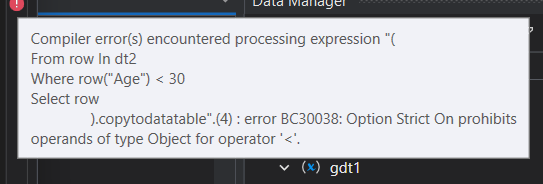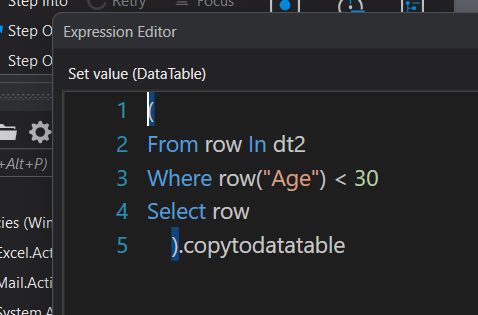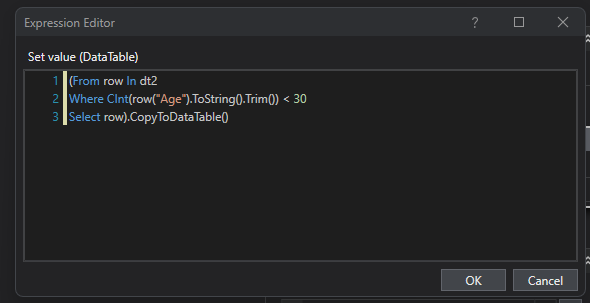# Linq helps

hi my datatable is having… Name age salary designation… so i want only those who are having age is less than 30… im using this linq but iam getting error. plz solve this and my erro isPlease try this LinQ query in the Assign activity:

`yourDt = yourDt.AsEnumerable.Where(Function(row) CInt(row("Age").ToString)<30).CopyToDataTable`

Hope this helps,
Best Regards.

You missed convert to int your row age, we can’t compare string agains int, Kindly try with

``````(From row In dt2
Where CInt(row("Age").ToString().Trim()) < 30
Select row).CopyToDataTable()
``````Regards!

Can you try this-

dtFiltered = dt.AsEnumerable().Where(Function(row) CInt(row(“age”)) < 30).CopyToDataTable()

Thanks!!

@katta_nikhil

You can actually use filter datatable activity directly to do this

If need only linq then you can try as below

`Dt.AsEnumerable.Where(function(x) Cint(x("Age").ToString)).CopyToDataTable`

We can use like this as well

`Dt.Select("[Age] < 30").CopyToDataTable`

Also it is better to first check the count before copytodatatable to check if atleast one row is returned

Hope this helps

Cheers

Hi @katta_nikhil ,
Convert to integer whilst comparing <,>,= with numbers
you can try this…
dt = (From row in dt2
Where CInt(row(“Age”).ToString.Trim) < 30
Select row).CopyToDataTable()